### 12 MyBinaryPowering

The basic idea of binary powering is to construct the result by considering the exponent in binary expansion. There are two methods: process the binary expansion of the exponent from left to right (from the highest to the lowest bit) or from right to left. The left-to-right version is very simple. We cite from [Knu69] and note that n has to be a positive integer.

Write n in the binary system (suppressing zeros at the left). Then replace each “1” by the pair of letters SX, replace each “0” by S, and cross off the “SX” which now appears at the left. The result is a rule for computing xn, if “S” is interpreted as the operation of squaring, and if “X” is interpreted as the operation of multiplying by x.

The right-to-left variant is a bit more complicated but more appropriate for our purpose. The main reason why we decide for the right-to-left variant is that for a destructive version we do not require the underlying domain to have a copy function.

82* 13+   58b  89
-------------------------------------------------------------------
----
---- ALLPROSE
---- Copyright (C) Ralf Hemmecke (ralf@hemmecke.de)
---- http://www.hemmecke.de/aldor
----
-------------------------------------------------------------------

#assert DontNeedLibraryMyAlps
#include "myalps"
pkg: MyBinaryPowering 84

Uses DontNeedLibraryMyAlps 37.

Type Constructor

Usage

x: X := binaryPower(67, 22, 7) \$ MyBinaryPowering(X, *\$X, N);
y: X := binaryPower(67, 22, 7) \$ MyBinaryPowering(X, +\$X, N);

Parameters

X: Type

The type of elements that should be exponentiated.

*: (X, X) -> X

An associative operation; for example, multiplication or addition on X.

N: BinaryRepresentedNumberType

A natural-number-like type for the exponents where

macro BinaryRepresentedNumberType == with {
zero?: % -> Boolean;
one?: % -> Boolean;
length: % -> MachineInteger;
bit?: (%, MachineInteger) -> Boolean;
}

We tacitly assume that no negative elements are passed as the third argument to binaryPower. In fact, there is no concept of negative here. Elements of N are only interpreted through their length and their bits. Typical candidates for N are Integer and MachineInteger.

Description

Binary exponentiation.

MyBinaryPowering provides an implementation of a binary exponentiation algorithm for the domain X with exponents in N. In fact, since the only requirement on the operation *: (X, X) -> X is associativity, there is no restriction of this package to just computing binaryPower(u,x,n) = uxn. For example, if X and N are Integer, then for the x and y from above it holds: x = 67 227 = 167121978496 and y = 67 + 22 7 = 221.

84pkg: MyBinaryPowering 84  (82)
MyBinaryPowering(
X: Type,
*: (X, X) -> X,
N: with {
zero?: % -> Boolean;
one?: % -> Boolean;
length: % -> MachineInteger;
bit?: (%, MachineInteger) -> Boolean;
}
): with {
exports: MyBinaryPowering 86
TRACEINSTANTIATION;
implementation: MyBinaryPowering 88
}

Defines:
MyBinaryPowering, used in chunk 89.

Uses TRACEINSTANTIATION 36.

Exports of MyBinaryPowering

binaryPower: (X, X, N) -> X Returns the first argument times the second argument raised to the power given by the third argument.

Export of MyBinaryPowering

binaryPower: (X, X, N) -> X

Usage

macro {
X == MachineInteger;
N == Integer;
}
import from MyBinaryPowering(X, * \$ X, N);
u: X := 1;
x: X := 22;
n: N := 7;
r: X := binaryPower(u, x, n);

Parameters

u: X

The element to multiply to the power.

x: X

The element to exponentiate.

n: N

The exponent.

Description

Returns the first argument times the second argument raised to the power given by the third argument.

binaryPower(u, x, n) computes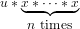by a binary powering algorithm where the operation *: (X, X) -> X can be any associative operation, i. e.,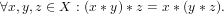Typical examples are multiplication and addition.

Remarks

The package MyBinaryPowering implements the function binaryPower in terms of a given function *: (X, X) -> X. The function * is allowed to destroy or reuse its first argument. If * is destructive then binaryPower destructively modifies its first and second argument. If * is non-destructive then binaryPower is non-destructive.

Also read the description of MyBinaryPowering about negative exponents, i. e., for negative values of elements of N.

86exports: MyBinaryPowering 86  (84)
binaryPower: (X, X, N) -> X;

In the following we are going to implement the right-to-left variant of the binary exponentiation algorithm, i. e., we process the exponent beginning with the lowest bit.

The implementation does not have access to a neutral element of the operation *: (X, X) -> X. In fact, there might not even exist a neutral element. Furthermore, if the operation *: (X, X) -> X works destructively on its first argument, we would need some additional storage anyway. That is the reason why we only provide a function with arguments u, x, n in order to compute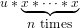instead of just giving the parameters x and n.

The algorithm given below certainly returns the correct result if n = 0 or n = 1.

For the correctness of the algorithm for n > 1 note that in the loop just after the statement

i := i + 1;

the invariant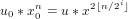(1)
holds where u0, x0, and n are the input parameters and u, x, and i are the values at that point of the computation. If i = s then n∕2i= 0 and consequently, u0 x0n = u.

Note that by the required signature of the package parameter N there is actually no direct way to really compute n∕2i. However, since N is considered in a binary representation, the value n∕2iis just the number n where the lowest i bits have been cut off.

Additionally note that we have used the short-hand xn to really mean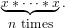88implementation: MyBinaryPowering 88  (84)
binaryPower(u: X, x: X, n: N): X == {
macro I == MachineInteger;
zero? n => u;
one? n  => u*x;
i: I := 0;
s: I := length n;
assert(s > 1);
repeat {
if bit?(n, i) then u := u * x;
i := i + 1;
i = s => return u;
x := x * x;
}
never;
}